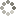# Daily 15 Up(No Ratings Yet)Loading...

Other 0

•GAME INSTRUCTIONSEvery day a new 15 up puzzle game in 4 sizes: 6x6, 7x7, 8x8 and 9x9. Use logic to solve the puzzle. Your goal is to create regions, where the numbers in each region total 15. The regions can be any shape, as long as the sum of the numbers in each region is 15. All the numbers must be used to complete the puzzle.

GAME DESCRIPTION

Every day a new 15 up puzzle game in 4 sizes: 6x6, 7x7, 8x8 and 9x9. Use logic to solve the puzzle. Your goal is to create regions, where the numbers in each region total 15. The regions can be any shape, as long as the sum of the numbers in each region is 15. All the numbers must be used to complete the puzzle.

RELATED GAMES